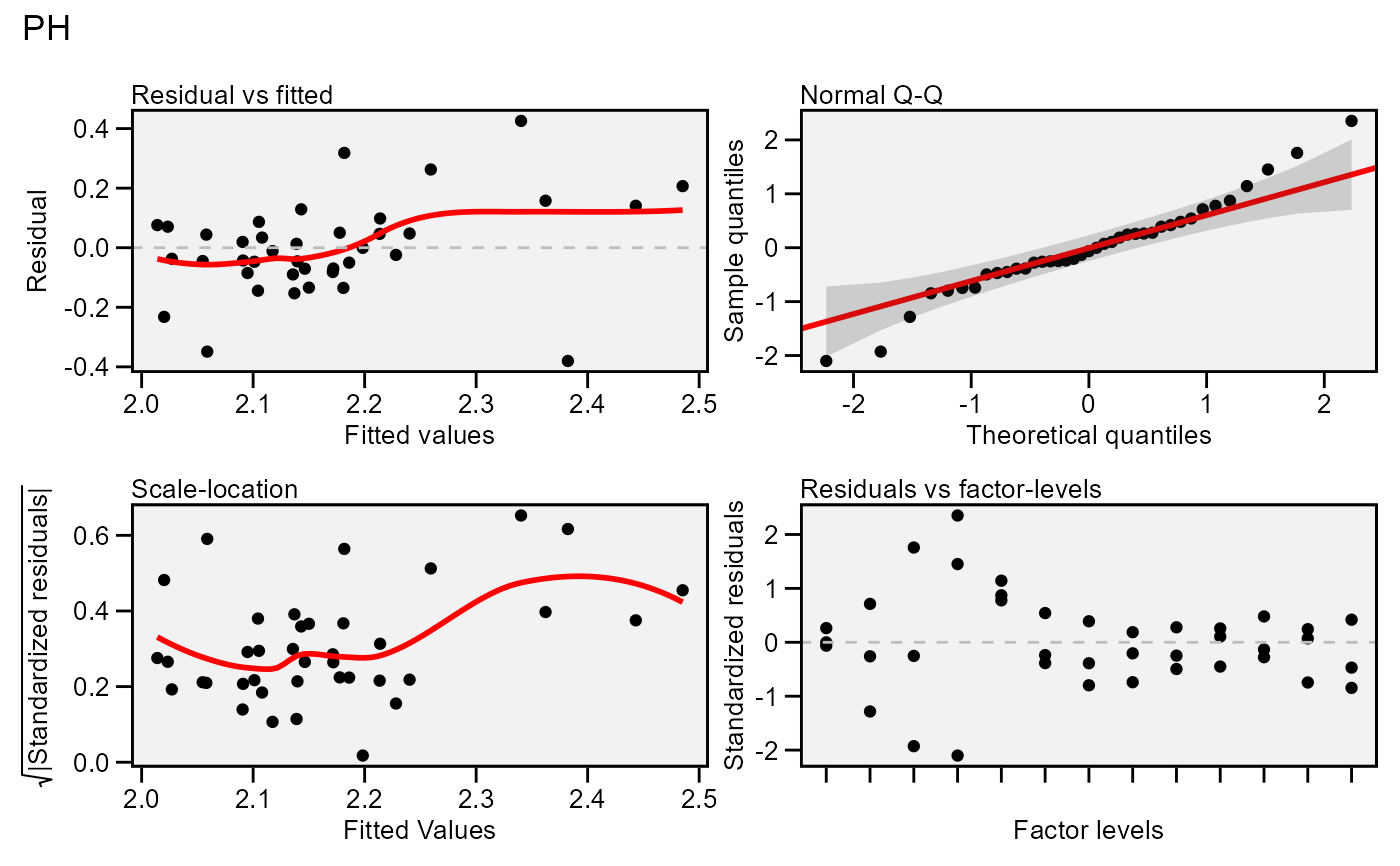Residual plots for a output model of class gamem. Six types of plots are produced: (1) Residuals vs fitted, (2) normal Q-Q plot for the residuals, (3) scale-location plot (standardized residuals vs Fitted Values), (4) standardized residuals vs Factor-levels, (5) Histogram of raw residuals and (6) standardized residuals vs observation order. For a waasb object, normal Q-Q plot for random effects may also be obtained declaring type = 're'

## Usage

# S3 method for gamem
plot(
x,
var = 1,
type = "res",
position = "fill",
rotate = FALSE,
conf = 0.95,
out = "print",
n.dodge = 1,
check.overlap = FALSE,
labels = FALSE,
plot_theme = theme_metan(),
alpha = 0.2,
fill.hist = "gray",
col.hist = "black",
col.point = "black",
col.line = "red",
col.lab.out = "red",
size.line = 0.7,
size.text = 10,
width.bar = 0.75,
size.lab.out = 2.5,
size.tex.lab = 10,
size.shape = 1.5,
bins = 30,
which = c(1:4),
ncol = NULL,
nrow = NULL,
...
)

## Arguments

x

An object of class gamem.

var

The variable to plot. Defaults to var = 1 the first variable of x.

type

One of the "res" to plot the model residuals (default), type = 're' to plot normal Q-Q plots for the random effects, or "vcomp" to create a bar plot with the variance components.

position

The position adjustment when type = "vcomp". Defaults to "fill", which shows relative proportions at each trait by stacking the bars and then standardizing each bar to have the same height. Use position = "stack" to plot the phenotypic variance for each trait.

rotate

Logical argument. If rotate = TRUE the plot is rotated, i.e., traits in y axis and value in the x axis.

conf

Level of confidence interval to use in the Q-Q plot (0.95 by default).

out

How the output is returned. Must be one of the 'print' (default) or 'return'.

n.dodge

The number of rows that should be used to render the x labels. This is useful for displaying labels that would otherwise overlap.

check.overlap

Silently remove overlapping labels, (recursively) prioritizing the first, last, and middle labels.

labels

Logical argument. If TRUE labels the points outside confidence interval limits.

plot_theme

The graphical theme of the plot. Default is plot_theme = theme_metan(). For more details, see ggplot2::theme().

alpha

The transparency of confidence band in the Q-Q plot. Must be a number between 0 (opaque) and 1 (full transparency).

fill.hist

The color to fill the histogram. Default is 'gray'.

col.hist

The color of the border of the the histogram. Default is 'black'.

col.point

The color of the points in the graphic. Default is 'black'.

col.line

The color of the lines in the graphic. Default is 'red'.

col.lab.out

The color of the labels for the 'outlying' points.

size.line

The size of the line in graphic. Defaults to 0.7.

size.text

The size for the text in the plot. Defaults to 10.

width.bar

The width of the bars if type = "contribution".

size.lab.out

The size of the labels for the 'outlying' points.

size.tex.lab

The size of the text in axis text and labels.

size.shape

The size of the shape in the plots.

bins

The number of bins to use in the histogram. Default is 30.

which

Which graphics should be plotted. Default is which = c(1:4) that means that the first four graphics will be plotted.

ncol, nrow

The number of columns and rows of the plot pannel. Defaults to NULL

...

Additional arguments passed on to the function patchwork::wrap_plots().

## Author

Tiago Olivoto tiagoolivoto@gmail.com

## Examples

# \donttest{
library(metan)
model <- gamem(data_g,
gen = GEN,
rep = REP,
resp = PH)
#> Evaluating trait PH |============================================| 100% 00:00:00

#> Method: REML/BLUP
#> Random effects: GEN
#> Fixed effects: REP
#> ---------------------------------------------------------------------------
#> P-values for Likelihood Ratio Test of the analyzed traits
#> ---------------------------------------------------------------------------
#>     model    PH
#>  Complete    NA
#>  Genotype 0.051
#> ---------------------------------------------------------------------------
#> Variables with nonsignificant Genotype effect
#> PH
#> ---------------------------------------------------------------------------
plot(model)
#> geom_smooth() using formula 'y ~ x'
#> geom_smooth() using formula 'y ~ x'# }